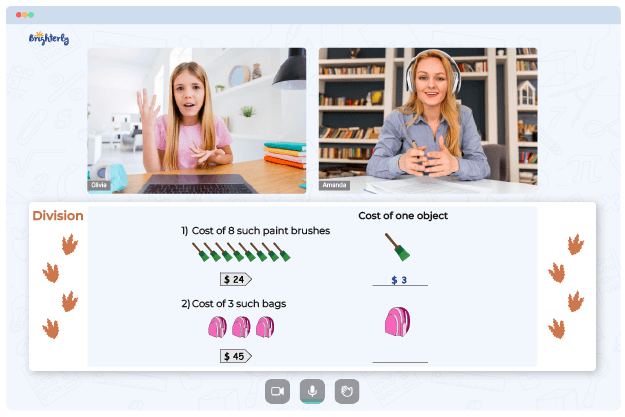# Least Common Multiple Worksheets

These least common multiple worksheets are created to help students to understand the concept of LCM and to solve problems using it. Before downloading our least common multiple worksheets with answer key, let’s find out what the least common multiple means, how to find it, and why it’s important in math.

## What is Least Common Multiple?

The least common multiple, abbreviated to LCM, is the lowest or smallest multiple a set of numbers have in common. To understand the concept of LCM, you’ll need to understand the meaning of multiples.

Multiples are the products you get when a number is multiplied by another number. Hence if you’re given three numbers and asked to find their lowest common multiple, you’ll need to list the multiples of each number, identify the multiples that all three numbers have in common, and choose the multiple with the lowest number.

Math for Kids

Is Your Child Struggling With Math?
1:1 Online Math TutoringYou can find the least common multiple of a group of numbers using different methods. The quickest way to do it is using the prime factorization method. When using this method, you’ll need to find the prime factor of the group of numbers and identify their least common multiple.

Another simple way to find LCM is the listing method explained above. The third way to find LCM is through the division method. In this method, you’ll need to divide the group of numbers by a common divisor until it can no longer divide the remainder. Then multiply the remainders and divisors to get the least common multiple.

## Benefits of Least Common Multiple Worksheet

These least common multiple worksheets will help students to understand least common multiples and how to solve problems using the different methods. Also, this worksheet comes with problems and answer keys that students can use to practice solving LCM questions. It also explains and gives a step-by-step guide on how to solve the problems using the different methods.### Least Common Multiple Worksheets PDF

Least Common Multiple Worksheet### Least Common Multiple Worksheets PDF

Least Common Multiple Worksheets 6Th Grade### Least Common Multiple Worksheets PDF

Least Common Multiples Worksheets 5Th Grade### Least Common Multiple Worksheets PDF

Finding Multiples Worksheet

You can download the least common multiple worksheets 6th grade for students in the 6th grade. There’s also a least common multiple worksheets 4th grade and a least common multiple worksheets 5th grade for students in the 4th and 5th grades.

### More Worksheets

Struggling with Division?• Start learning with an online tutor.

Is your child finding it challenging to understand division concepts? An online tutor could be the solution.